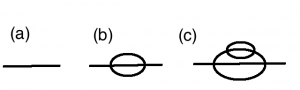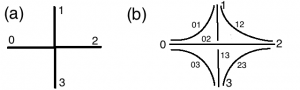In statistical physics, one can imagine a model where one parameter is not fixed, but instead is random. We say that the disorder is

• Quenched if the random parameters do not evolve with time, they are chosen randomly at the beginning of time but then stay constant during the evolution of the system
• Annealed if the random parameters can evolve with time.

Recently, a model with quenched disorder has become a very hot topic in theoretical physics, the SYK model. Those letters stand for Sachdev and Ye who introduced the model in the early '90 in statistical physics, and Kitaev who used the model in a series of talks last year at KITP as a model of quantum holography. Kitaev seemingly didn't find it useful to write a paper, so people have to cite this talk, which fortunately has been registered (this was not the case, for instance, for Witten's talk at Strings 1995). The model is a quantum mechanical system (this means that the fields evolve in $1+0$ dimensions) of $N$ real fermions. In the simplest setup, the interaction is $$j_{i_1 i_2 i_3 i_4}\psi_{i_1}\psi_{i_2}\psi_{i_3}\psi_{i_4}$$ where the couplings $j_{i_1 i_2 i_3 i_4}$ are Gaussian quenched random variables with $$\langle j_{i_1 i_2 i_3 i_4} j_{i'_1 i'_2 i'_3 i'_4} \rangle \sim \delta_{i_1 i'_1}\delta_{i_2 i'_2}\delta_{i_3 i'_3}\delta_{i_4 i'_4} \frac{ J^2}{N^3}$$

This model is interesting for at least three reasons (see here for a detailed discussion, and also these CERN winter school lectures by Douglas Stanford that I was lucky enough to attend):

1. It develops an approximate conformal symmetry in the IR, which is the holographic dual of gravity with very near extremal black holes.
2. It has an underlying chaotic dynamics, which is maximal in a certain sense (it saturates the Maldacena-Shenker-Stanford bound on the Lyapunov exponent that appears in out-of-time-order correlation functions)
3. The theory is solvable in the large $N$ limit even at finite temperature, which is not the case of $\mathcal{N}=4$ SYM, for instance.

The fact that the theory simplifies drastically in the large $N$ limit can be reduced to the structure of Feynman diagrams that survive in this limit, than can be constructed iteratively as follows:This picture is taken from a very recent paper by Edward Witten in which another model is proposed that would share the same structure of Feynman diagrams in a large $N$ limit, but with no quenched disorder. This model belongs to the big family of tensor models (see for instance this overview by Razvan Gurau). The fields are four fermions $\psi_{0,1,2,3}$ that belong to representations of a big gauge group $G$. This gauge group is, up to a discrete quotient, isomorphic to $O(n)^6$, where the is one factor $O(n)$ for each edge of a tetrahedron with vertices labeled $0,1,2,3$. The field $\psi_i$ transforms as a vector with $n$ components for each $O(n)$ that sits on an edge reaching $\psi_i$, so it is a tensor of size $n^3$. The Hamiltonian is very simple, $$H \sim \frac{J}{n^{3/2}} \psi_0 \psi_1 \psi_2 \psi_3$$ where the indices are contracted in an appropriate way. One can then show that in the large $n$ limit, only the diagrams as pictured above survive. Now, each line in those diagrams can be treated as three strands (much as Feynman diagrams become ribbon diagrams for matrix models), and a standard counting in powers of $n$, that appear for every closed strand loop gives the result. The contraction of indices in the Hamiltonian is such that looking at those strands, the vertex looks like the following, again taken from Witten's paper:In this framework, then, quenched disorder has been eliminated, and the interesting property number 3 above is preserved, thanks to the structure of the Feynman diagrams. What about the two other features that make the SYK model so interesting? Well, Witten argues that

The average of a quantum system over quenched disorder is not really a quantum system, so the reliance on quenched disorder might make it difficult to apply the SYK model to some subtle questions about black holes.

In fact, the good quantum system proposed here would then appear to be even more appropriate to gravitational physics. On the matter of chaos, I don't know at this stage where Witten's model stands...

References

• An SYK-Like Model Without Disorder, Edward Witten. arXiv:1610.09758.
• Comments on the Sachdev-Ye-Kitaev model, Juan Maldacena, Douglas Stanford. arXiv:1604.07818
• The Spectrum in the Sachdev-Ye-Kitaev Model, Joseph Polchinski, Vladimir Rosenhaus. arXiv:1601.06768